CHEMICAL EQUATIONS REACTION STOICHIOMETRY Chapter 3 Chemical Equations

• Slides: 29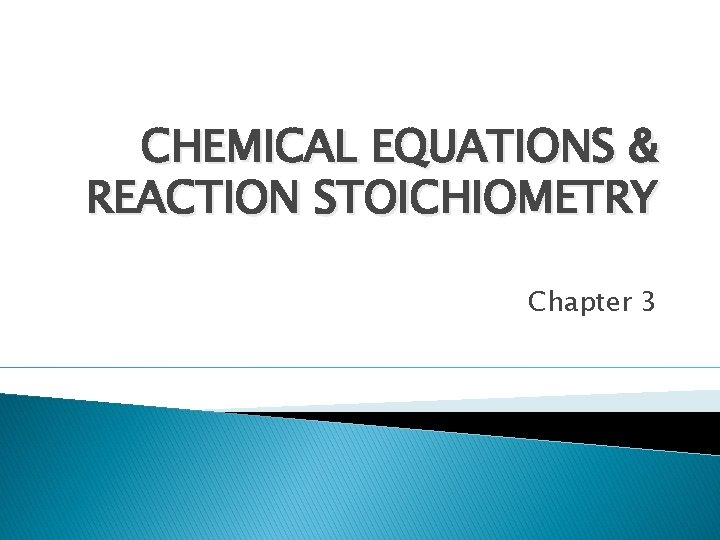CHEMICAL EQUATIONS & REACTION STOICHIOMETRY Chapter 3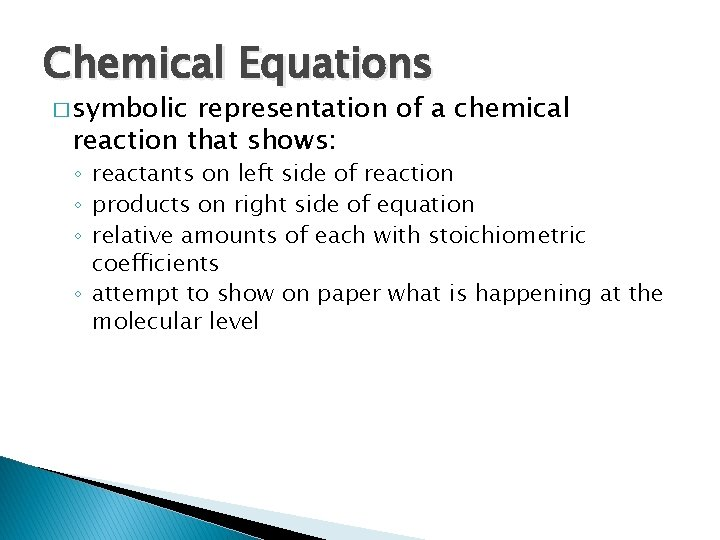Chemical Equations � symbolic representation of a chemical reaction that shows: ◦ reactants on left side of reaction ◦ products on right side of equation ◦ relative amounts of each with stoichiometric coefficients ◦ attempt to show on paper what is happening at the molecular level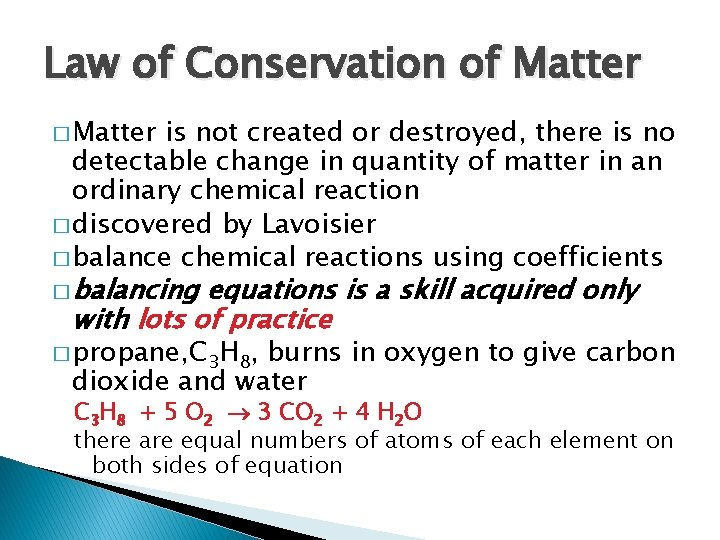Law of Conservation of Matter � Matter is not created or destroyed, there is no detectable change in quantity of matter in an ordinary chemical reaction � discovered by Lavoisier � balance chemical reactions using coefficients � balancing equations is a skill acquired only with lots of practice � propane, C 3 H 8, burns in oxygen to give carbon dioxide and water C 3 H 8 + 5 O 2 ® 3 CO 2 + 4 H 2 O there are equal numbers of atoms of each element on both sides of equationCalculations Based on Chemical Equations � Ex. 1) How many CO molecules are required to react with 27 formula units of Fe 2 O 3 to make iron and carbon dioxide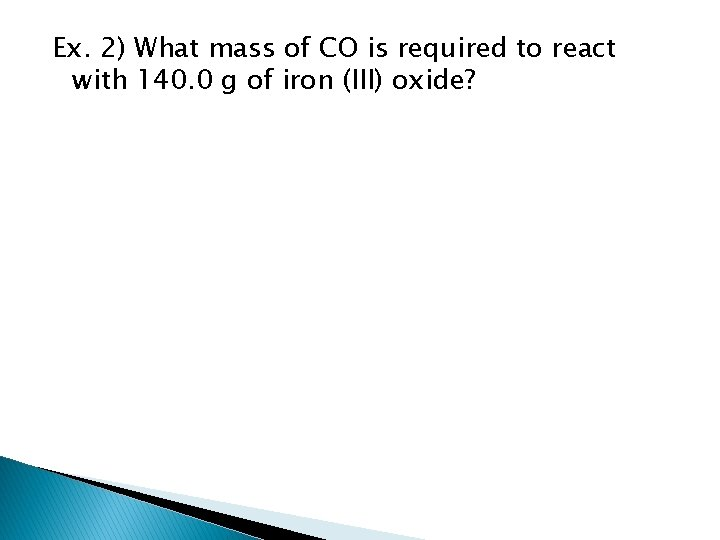Ex. 2) What mass of CO is required to react with 140. 0 g of iron (III) oxide?� Ex. 3) How many pounds of carbon monoxide would react with 128 pounds of iron (III) oxide?Limiting Reactant (Reagent) Concept � Most experiments do not have the exact amounts needed to completely react. LR helps us decide which reactant is in excess (the one we have plenty of) and which reactant is limiting (the one that will determine the outcome of the reaction) � ~ If a box has 87 bolts, 113 washers and 99 nuts, how many sets consisting of one bolt, two washers and one nut can you make?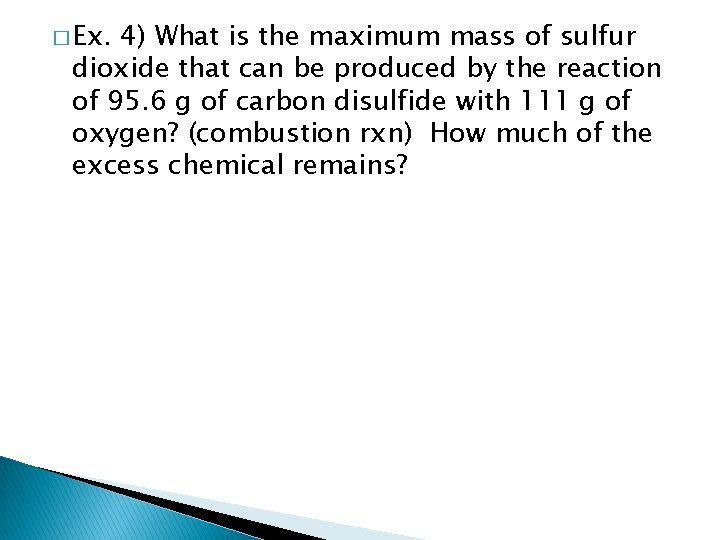� Ex. 4) What is the maximum mass of sulfur dioxide that can be produced by the reaction of 95. 6 g of carbon disulfide with 111 g of oxygen? (combustion rxn) How much of the excess chemical remains?Percent Yields from Reactions � Most rxns in real life do not go to completion. A few reasons why ◦ Reactants did not react 100% to give the products ◦ Side reactions can occur to form undesirable products as well as the wanted products � theoretical yield is what we have been calculating on paper � actual yield is what you have made in the lab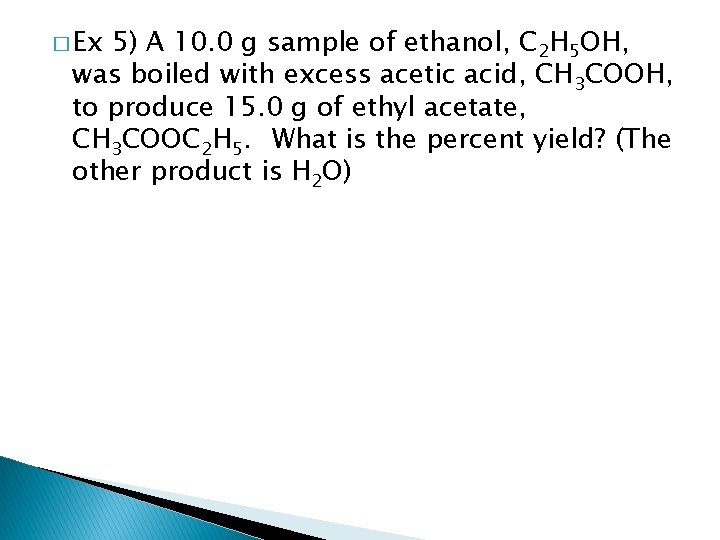� Ex 5) A 10. 0 g sample of ethanol, C 2 H 5 OH, was boiled with excess acetic acid, CH 3 COOH, to produce 15. 0 g of ethyl acetate, CH 3 COOC 2 H 5. What is the percent yield? (The other product is H 2 O)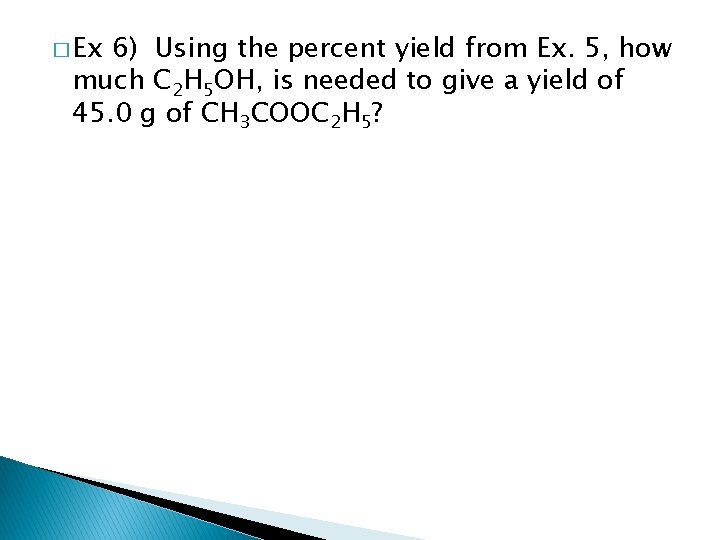� Ex 6) Using the percent yield from Ex. 5, how much C 2 H 5 OH, is needed to give a yield of 45. 0 g of CH 3 COOC 2 H 5?Sequential Reactions � Sequential ~ multiple steps are needed for a rxn to go to completion HNO 3 Sn C 6 H 6 C 6 H 5 NO 2 C 6 H 5 NH 2 H 2 SO 4 Conc HCl� Ex. 7) Starting with 100. g of benzene (C 6 H 6), calculate theoretical yield of nitrobenzene (C 6 H 5 NO 2) and of aniline (C 6 H 5 NH 2). (1 to 1 mole ratios)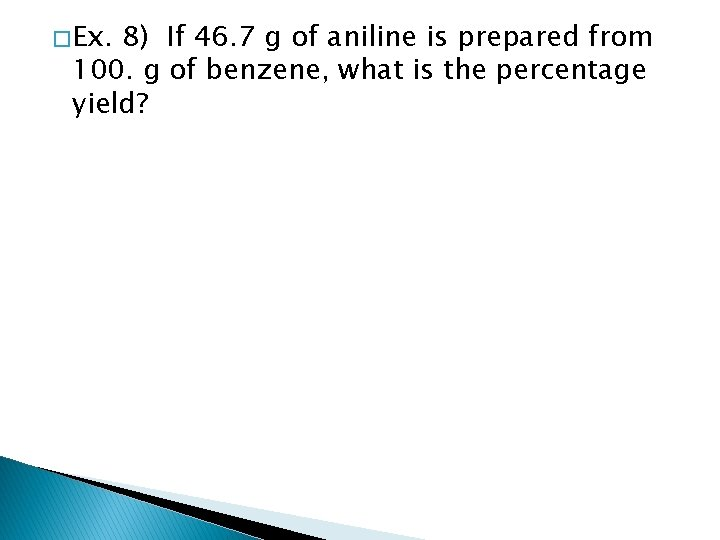� Ex. 8) If 46. 7 g of aniline is prepared from 100. g of benzene, what is the percentage yield?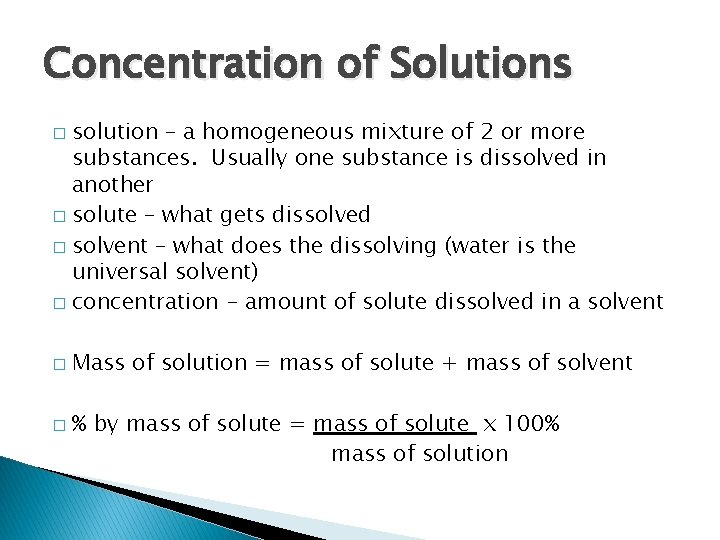Concentration of Solutions solution – a homogeneous mixture of 2 or more substances. Usually one substance is dissolved in another � solute – what gets dissolved � solvent – what does the dissolving (water is the universal solvent) � concentration - amount of solute dissolved in a solvent � � � Mass of solution = mass of solute + mass of solvent % by mass of solute = mass of solute x 100% mass of solution� Ex. 9) Calculate the mass of potassium nitrate required to make 277 g of solution that is 20. 0% KNO 3 by mass. What is the mass of water in the solution?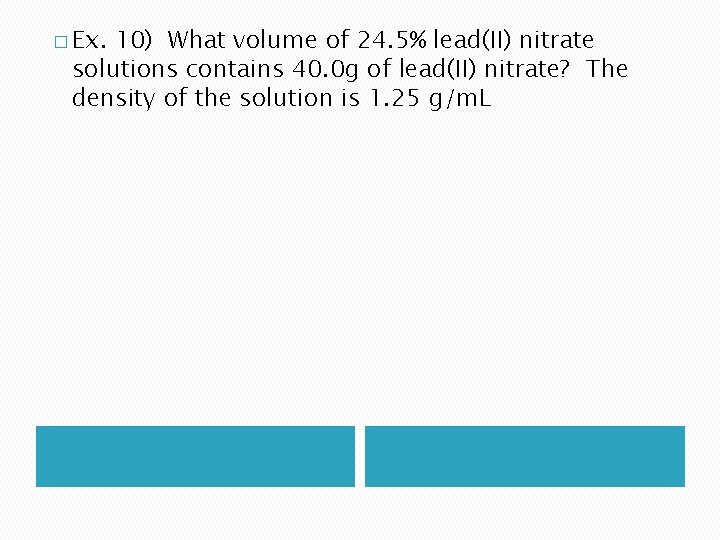� Ex. 10) What volume of 24. 5% lead(II) nitrate solutions contains 40. 0 g of lead(II) nitrate? The density of the solution is 1. 25 g/m. L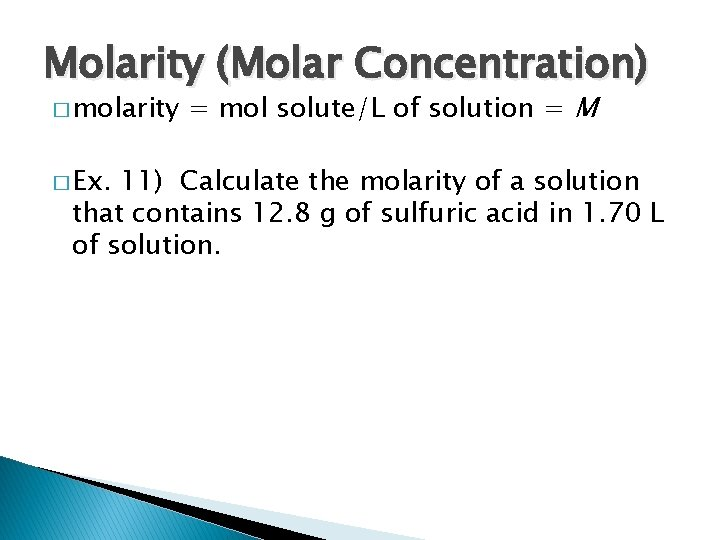Molarity (Molar Concentration) � molarity � Ex. = mol solute/L of solution = M 11) Calculate the molarity of a solution that contains 12. 8 g of sulfuric acid in 1. 70 L of solution.� Ex. 12) Determine the mass of calcium nitrate required to prepare 3. 55 L of 0. 822 M solution.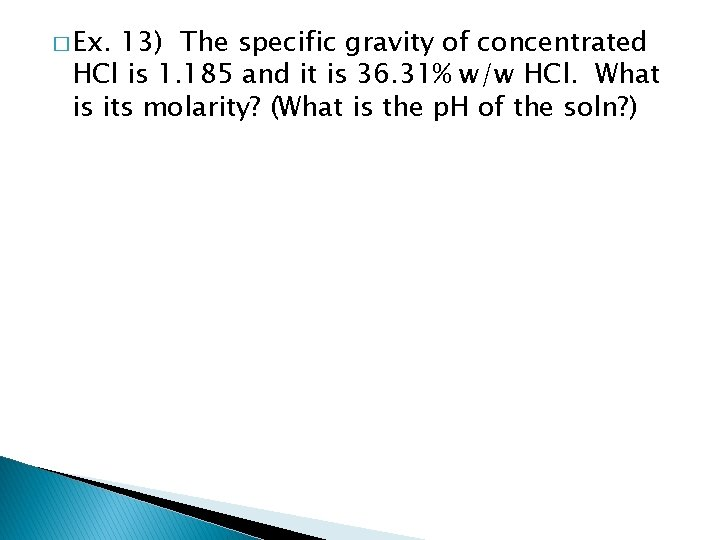� Ex. 13) The specific gravity of concentrated HCl is 1. 185 and it is 36. 31% w/w HCl. What is its molarity? (What is the p. H of the soln? )Dilution of Solutions number of moles of solute remains constant � M 1 V 1 = M 2 V 2 works because # of moles is constant � If 10. 0 m. L of 12 M HCl is added to enough water to give 100. m. L of solution, what is concentration of the new solution? �� Ex. 14) What volume of 18. 0 M sulfuric acid is required to make 2. 50 L of a 2. 44 M sulfuric acid solution? M 1 V 1 = M 2 V 2 n 1 n 2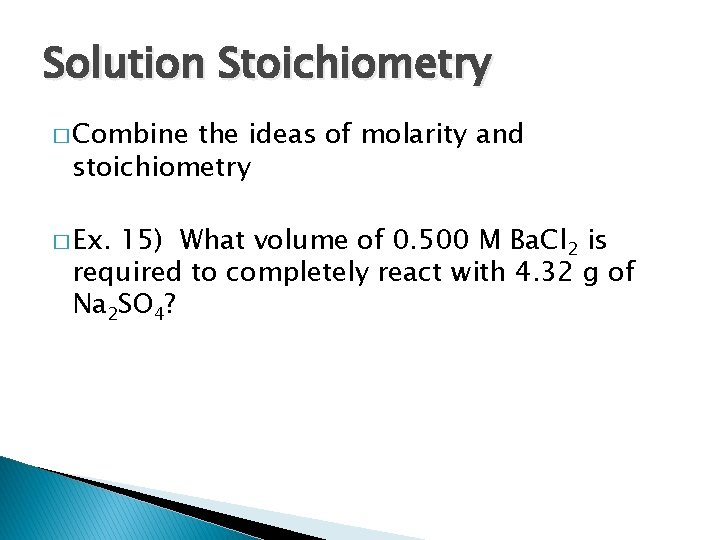Solution Stoichiometry � Combine the ideas of molarity and stoichiometry � Ex. 15) What volume of 0. 500 M Ba. Cl 2 is required to completely react with 4. 32 g of Na 2 SO 4?� Ex. 16) a) What volume of 0. 220 M Na. OH will react with 50. 0 m. L 0 f 0. 220 M aluminum nitrate? b) What mass of aluminum hydroxide precipitates?Titrations � method of determining the concentration of solutions once the concentration of a standard solution is known � requires special glassware� Ex. 17) What is the molarity of a barium hydroxide solution if 44. 4 m. L of 0. 103 M HCl is required to react with 38. 8 m. L of the barium hydroxide solution?� In the lab, acid-base rxns are carried out as a titration: ◦ Standard solution: a soln of known concentration ◦ Standardization: process by which the conc. of a standard soln is determined. ◦ Equivalence point: point in a titration at which chemically equivalent amounts of reactants have reacted ◦ Endpoint: point in a titration where the indicator changes color and the titration is stopped.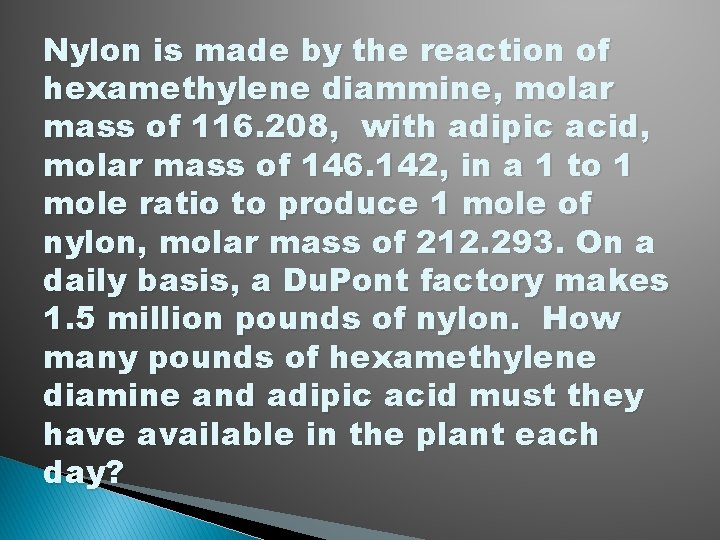Nylon is made by the reaction of hexamethylene diammine, molar mass of 116. 208, with adipic acid, molar mass of 146. 142, in a 1 to 1 mole ratio to produce 1 mole of nylon, molar mass of 212. 293. On a daily basis, a Du. Pont factory makes 1. 5 million pounds of nylon. How many pounds of hexamethylene diamine and adipic acid must they have available in the plant each day?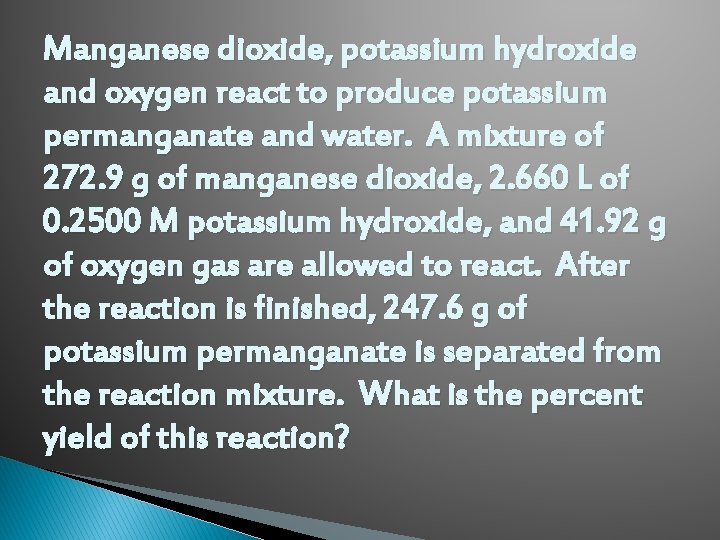Manganese dioxide, potassium hydroxide and oxygen react to produce potassium permanganate and water. A mixture of 272. 9 g of manganese dioxide, 2. 660 L of 0. 2500 M potassium hydroxide, and 41. 92 g of oxygen gas are allowed to react. After the reaction is finished, 247. 6 g of potassium permanganate is separated from the reaction mixture. What is the percent yield of this reaction?